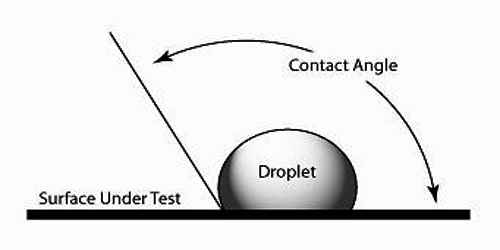Physics

# When Angle of Contact is different for different points of Solid and Liquid?Angle of contact is different for different points of solid and liquid

The angle which the tangent to the liquid surface at the point of contact makes with the solid surface inside the liquid is called the angle of contact. The angle of contact is always measured through the liquid. It is denoted as θ or α.

When a liquid comes in contact with a solid surface it is generally curved at the point of contact. There exists an angle between these two contact surfaces. Apparently, this angle is the contact angle.

The angle of contact is different for different points of solid and liquid. For ordinary water and glass, the angle of contact is nearly 8°, whereas, for mercury and glass, it is 1350.

(1) lf θ < 900 i.e., acute angle of contact, then the following characteristics are observed:

• The surface of liquid in the capillary tube will be concave.
• The liquid will rise in the capillary tube.
• The liquid will wet the wall of the tube.
• The adhesive force is greater than the cohesive force.

(ii) If θ > 900 i.e., the obtuse angle of contact, then the following characteristics are found:

• The surface of the liquid will be comvex.
• The liquid will fall in a capillary tube.
• The liquid will not wet the wall of the tube
• Cohesive force is greater than the adhesive Force.